# Class 9 RD Sharma Solutions – Chapter 23 Graphical Representation of Statistical Data – Exercise 23.2 | Set 1

• Last Updated : 18 Mar, 2021

### Question 1. Explain the reading and interpretation of bar graphs.

Solution:

A bar graph comprises a sequence of vertical or horizontal bar lines, also known as rectangles which may be either horizontal or vertical in nature. It is used to simulate the collection of the information over a period of time. Also, it concludes various observations over a time frame from a given bar graph to specify interpretation of the bar graph.

### Question 2. Read the following bar graph and answer the following questions:

(i) What information is given by the bar graph?

(ii) In which year the export is minimum?

(iii) In which year the import is maximum?

(iv) In which year the difference of the values of export and import is maximum?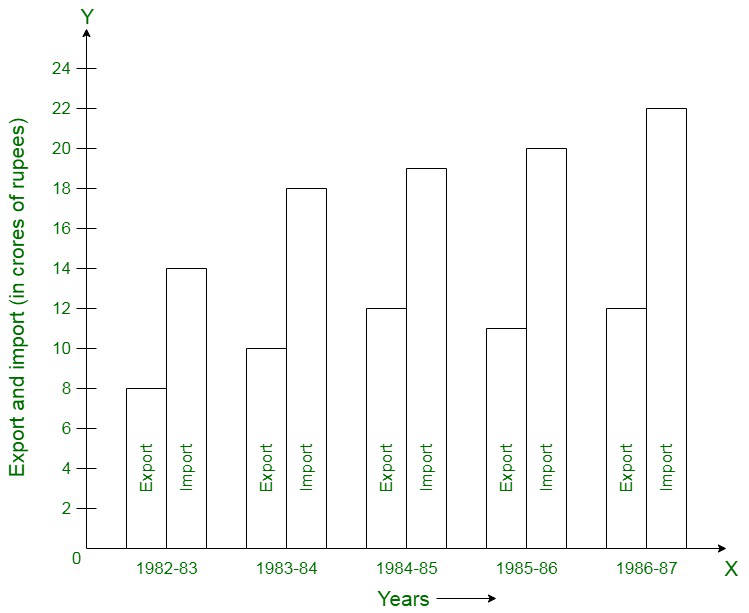Solution:

(i) The specified bar graph depicts the import and export (specified in 100 Crores of rupees) from 1982-83 to 1986-87.

(ii) 1982-83

(iii) 1986-87

(iv) 1986-87

### Question 3. The following bar graph shows the results of an annual examination in a secondary school. Read the bar graph given below, and choose the correct alternative in each of the following: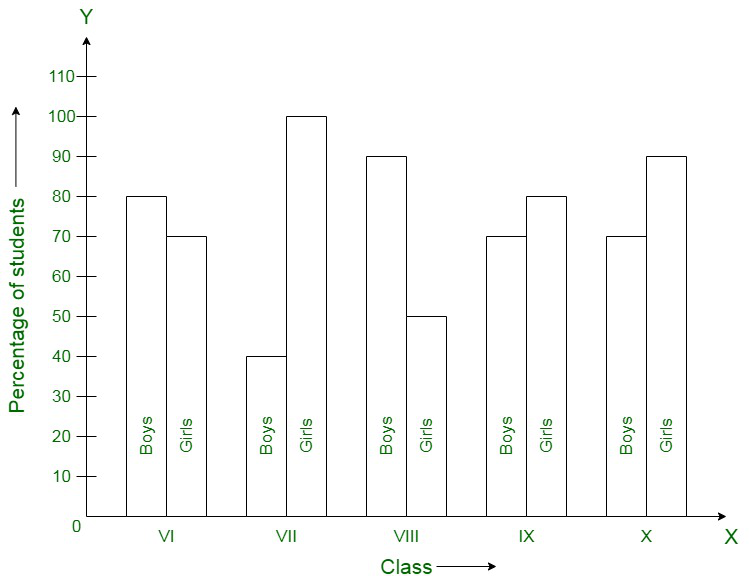(i) The pair of classes in which the results of boys and girls are inversely proportional are:

(a) VI, VIII (b) VI, IX (c) VII, IX (d) VIII, X

(ii) The class having the lowest failure rate of girls is:

(a) VI (b) X (c) IX (d) VIII

(iii) The class having the lowest pass rate of students is:

(a) VI (b) VII (c) VIII (d) IX

Solution:

(i) Here, the option (b) is correct.

(ii) Here, the option (a) is correct.

(iii) Here, the option (b) is correct.

The sum of the corresponding heights of the bars for boys and girls in class VII = 95 + 40 = 135 [minimum]

### Construct a bar graph to represent the above data.

Solution:

The following bar graph represents the bars where the horizontal and vertical axes represent the years and the number of applicants registered (in thousands) respectively.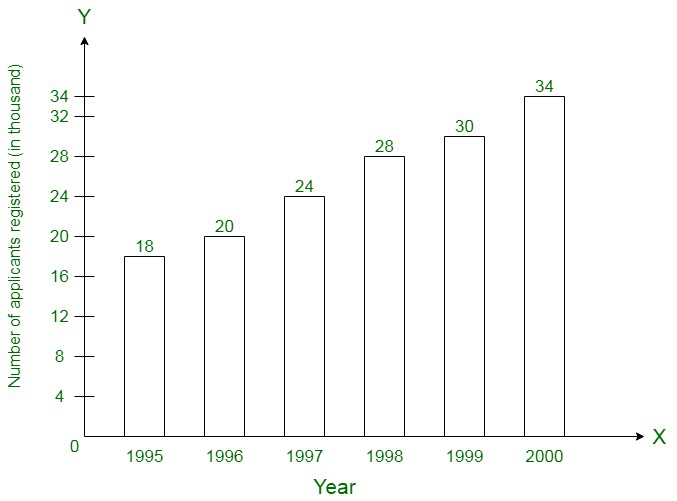### Construct a bar graph to represent the above data on a graph paper by using the scale 1 big divisions = 20 thousand tonnes.

Solution:

The following bar graph represents the bars where the horizontal and vertical axes represent the plants and the production in a thousand tonnes respectively.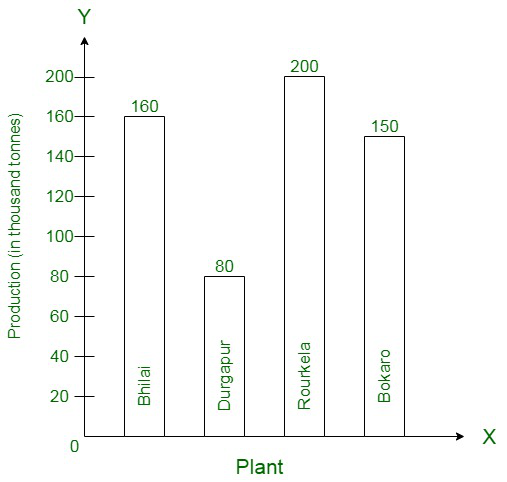### Represent the above data with the help of a bar graph.

Solution:

The following bar graph represents the bars where the horizontal and vertical axes represent the years and the route lengths in a thousand km respectively.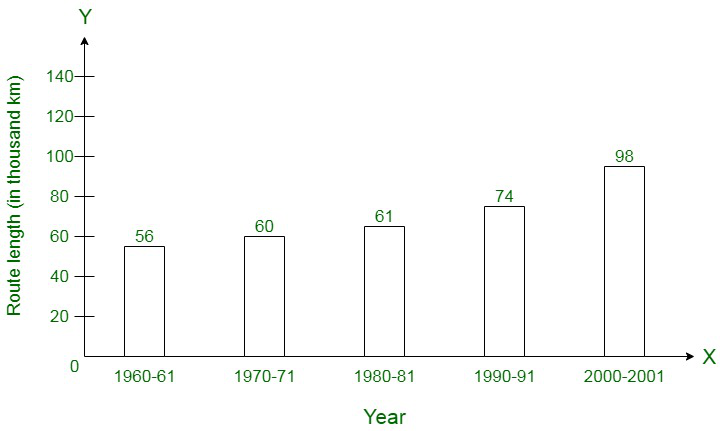### Question 7. The following data gives the amount of loans (in crores of rupees) disbursed by a bank during some years:

(i) Represent the above data with the help of a bar graph.

(ii) With the help of the bar graph, indicate the year in which amount of loan is not increased over that of the preceding year.

Solution:

(i)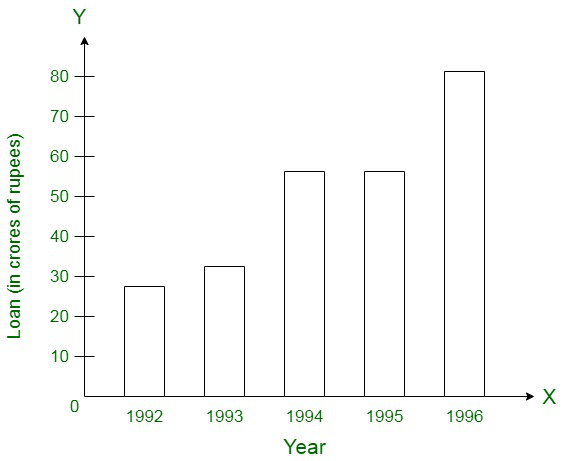(ii) In 995, the loan is not increased over that period of the preceding year.

### Draw the bar graph to represent the above information.

Solution:

The following bar graph represents the bars where the horizontal and vertical axes represent the years and the interest amount in lakhs respectively.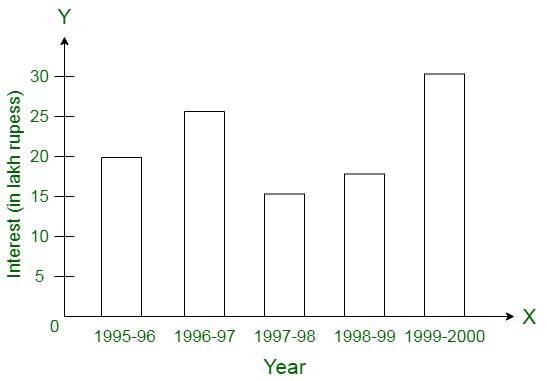### Represent the above information by a bar graph.

Solution:

The following bar graph represents the bars where the horizontal and vertical axes represent the countries and the average age(in years) respectively.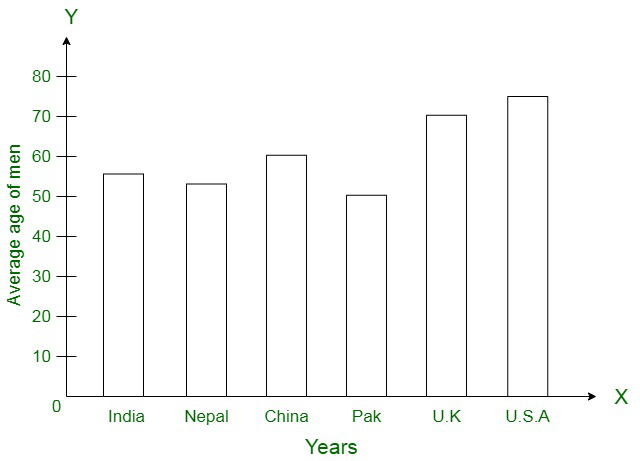### Represent the above data with the help of a bar graph.

Solution:

The following bar graph represents the bars where the horizontal and vertical axes represent the years and the production (in a thousand tonnes) respectively.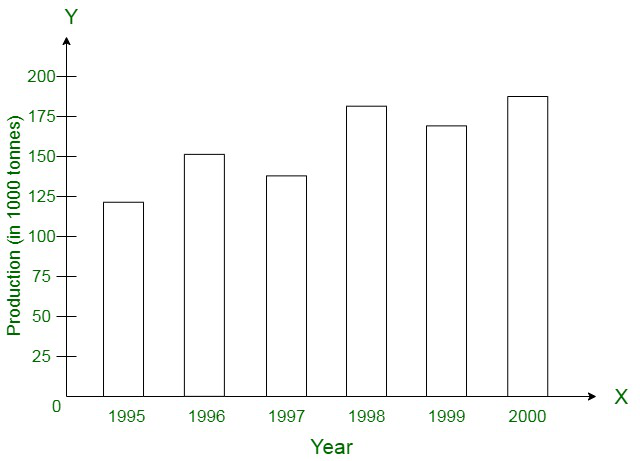My Personal Notes arrow_drop_up